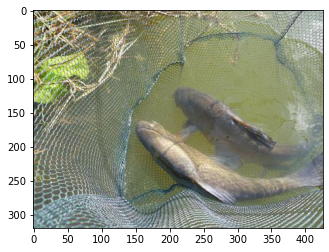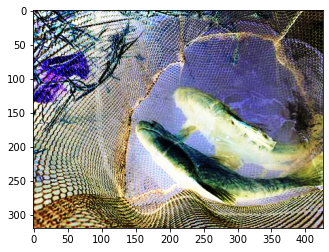In this tutorial, we will be looking at how one can make use of AutoAugment as a data augmentation technique to train a neural net.

We look at:

1. How can we use the timm training script to apply AutoAugment.
2. How can we use AutoAugment as a standalone data augmentation technique for custom training loops.
3. Take a deep-dive inside the source code of AutoAugment.

## AutoAugment using the timm's training script

To train a model using the timm's and apply auto augmentation data policy, simply add the --aa flag with a value of 'original' or 'v1' like so:

python train.py ../imagenette2-320 --aa original


The above script trains a neural net using AutoAugment as an augmentation technique with the same policies as mentioned in the paper.

## AutoAugment as a standalone data augmentation technique for custom training loops

In this section we will see how we can use AutoAugment as a standalone data augmentation technique in our own custom training loops.

We can simply create a transform function called tfm using the auto_augment_transform function in timm. We pass in a config_str and some hparams to the function to create our transform function.

Below, we create our transformation function tfm, and also creat an input image X which is the image of a "tench" as used everywhere else in this documentation.

from timm.data.auto_augment import auto_augment_transform
from PIL import Image
from matplotlib import pyplot as plt

tfm = auto_augment_transform(config_str = 'original', hparams = {'translate_const': 100, 'img_mean': (124, 116, 104)})
X   = Image.open('../../imagenette2-320/train/n01440764/ILSVRC2012_val_00000293.JPEG')
plt.imshow(X);Having visualized X, let's apply the transform function which applies the auto-augmentation policy to X and let's visualize the results below:

plt.imshow(tfm(X));As we can see, the function tfm applies auto augmentation technique to the input image X.

Thus, as long as we make sure that this function transforms an input image that is of type PIL.Image, we can use timm to apply AutoAugment in our custom training loops.

## timm's implementation of AutoAugment

Let's now take a deep dive to understand the timm's implementation of AutoAugment policy.

### auto_augment_transform

The complete source code of the auto_augment_transform that we used above looks like:

def auto_augment_transform(config_str, hparams):
"""
Create a AutoAugment transform

:param config_str: String defining configuration of auto augmentation. Consists of multiple sections separated by
dashes ('-'). The first section defines the AutoAugment policy (one of 'v0', 'v0r', 'original', 'originalr').
The remaining sections, not order sepecific determine
'mstd' -  float std deviation of magnitude noise applied
Ex 'original-mstd0.5' results in AutoAugment with original policy, magnitude_std 0.5

:param hparams: Other hparams (kwargs) for the AutoAugmentation scheme

:return: A PyTorch compatible Transform
"""
config = config_str.split('-')
policy_name = config
config = config[1:]
for c in config:
cs = re.split(r'(\d.*)', c)
if len(cs) < 2:
continue
key, val = cs[:2]
if key == 'mstd':
# noise param injected via hparams for now
hparams.setdefault('magnitude_std', float(val))
else:
assert False, 'Unknown AutoAugment config section'
aa_policy = auto_augment_policy(policy_name, hparams=hparams)
return AutoAugment(aa_policy)


This is very similar to RandAugment's implementation. Basically, we pass in a config string, and based on the config string, this function sets up some hparams that then get passed to auto_augment_policy to create the policy. Finally we wrap this aa_policy around the AutoAugment class which get's returned to be applied to the input data.

Let's take a look inside the auto_augment_policy and AutoAugment source code below.

### auto_augment_policy

The source code of the auto_augment_policy function looks something like:

def auto_augment_policy(name='v0', hparams=None):
hparams = hparams or _HPARAMS_DEFAULT
if name == 'original':
return auto_augment_policy_original(hparams)


Basically, this function accepts a policy name and then returns the corresponding augmentation policy.

Let's take a look inside the auto_augment_policy_original function below.

### auto_augment_policy_original

The source code of this function looks like:

def auto_augment_policy_original(hparams):
# ImageNet policy from https://arxiv.org/abs/1805.09501
policy = [
[('PosterizeOriginal', 0.4, 8), ('Rotate', 0.6, 9)],
[('Solarize', 0.6, 5), ('AutoContrast', 0.6, 5)],
[('Equalize', 0.8, 8), ('Equalize', 0.6, 3)],
[('PosterizeOriginal', 0.6, 7), ('PosterizeOriginal', 0.6, 6)],
[('Equalize', 0.4, 7), ('Solarize', 0.2, 4)],
[('Equalize', 0.4, 4), ('Rotate', 0.8, 8)],
[('Solarize', 0.6, 3), ('Equalize', 0.6, 7)],
[('PosterizeOriginal', 0.8, 5), ('Equalize', 1.0, 2)],
[('Rotate', 0.2, 3), ('Solarize', 0.6, 8)],
[('Equalize', 0.6, 8), ('PosterizeOriginal', 0.4, 6)],
[('Rotate', 0.8, 8), ('Color', 0.4, 0)],
[('Rotate', 0.4, 9), ('Equalize', 0.6, 2)],
[('Equalize', 0.0, 7), ('Equalize', 0.8, 8)],
[('Invert', 0.6, 4), ('Equalize', 1.0, 8)],
[('Color', 0.6, 4), ('Contrast', 1.0, 8)],
[('Rotate', 0.8, 8), ('Color', 1.0, 2)],
[('Color', 0.8, 8), ('Solarize', 0.8, 7)],
[('Sharpness', 0.4, 7), ('Invert', 0.6, 8)],
[('ShearX', 0.6, 5), ('Equalize', 1.0, 9)],
[('Color', 0.4, 0), ('Equalize', 0.6, 3)],
[('Equalize', 0.4, 7), ('Solarize', 0.2, 4)],
[('Solarize', 0.6, 5), ('AutoContrast', 0.6, 5)],
[('Invert', 0.6, 4), ('Equalize', 1.0, 8)],
[('Color', 0.6, 4), ('Contrast', 1.0, 8)],
[('Equalize', 0.8, 8), ('Equalize', 0.6, 3)],
]
pc = [[AugmentOp(*a, hparams=hparams) for a in sp] for sp in policy]
return pc


It's actually pretty simple the way auto_augment_policy_originalhas been constructed. As we know from the paper, the ImageNet policy consits of 25 sub policies. We iterate through the policy above to get a sub-policy sp. Finally we also iterate through each operation referred to as a in sub-policy sp and wrap it around the AugmentOp class.

We have already looked into the AugmentOp class here.

Thus, in essence, each operation in the sub-policy get's converted to an instance of AugmentOp class based on the probability and magnitude values mentioned in the policy above. This becomes the policy that get's returned to create aa_policy in auto_augment_transform.

## AutoAugment

As a last step in auto_augment_transform we wrap this aa_policy in AutoAugment class and that's what get's applied to the input data. So let's look into AutoAugment below:

class AutoAugment:

def __init__(self, policy):
self.policy = policy

def __call__(self, img):
sub_policy = random.choice(self.policy)
for op in sub_policy:
img = op(img)
return img


Really, this is one of the simplest implementations of AutoAugment. Similar to the paper, we select a random sub-policy, which consists of two operations (where each operation consists of an augmentation function, magnitude and probability of applying the augmentation function), and finally apply to these operations to the img to return the augmented image.